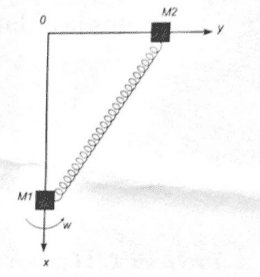# Solve a system of two linked harmonic oscillators

YellowBiro
Thread moved from the technical forums, so no Homework Template is shown
$$m_1 \ddot{x} - m_1 g + \frac{k(d-l)}{d}x=0$$
$$m_2 \ddot{y} - m_2 \omega^2 y + \frac{k(d-l)}{d}y=0$$

It is two masses connected by a spring. ##d=\sqrt{x^2 + y^2}## and ##l## is the length of the relaxed spring (a constant).

What is the strategy to solve such a system? I tried substituting one in the other and got

$$y m_1\ddot{x} -m_1gy=xm_2\ddot{y}-m_2\omega^2 yx$$

I don't know how to continue from here. Can you maybe keep one coordinate constant and solve for the other? Doesn't seem to make much sense.

Also the question in the exam says, "Determine the two equilibrium solutions" but I presume, you first have to find the solutions first, right?

Could you please give a diagram showing the coordinate system, the spring, etc., with the different symbols marked in the diagram?

YellowBiro
Could you please give a diagram showing the coordinate system, the spring, etc., with the different symbols marked in the diagram?Homework Helper
See nothing ! Just anLast edited:
YellowBiro
Homework Helper
Yes. Now I seeand a whole lot of imgur crud. The equations make a little more sense now.

I presume, you first have to find the solutions
You assume wrongly. Try to solve ##x## and ##y## from ##\ \ \ddot x = 0 \land \ddot y = 0##

#### Attachments

YellowBiro
Yes. Now I see
View attachment 219023
and a whole lot of imgur crud. The equations make a little more sense now.

You assume wrongly. Try to solve ##x## and ##y## from ##\ \ \ddot x = 0 \land \ddot y = 0##

Oh I see now. I actually got that the accelerations are zero from the Hamiltonian. So then if I integrate I get two solutions which I then substitute in the eom above and end up with two equations and two unknowns.